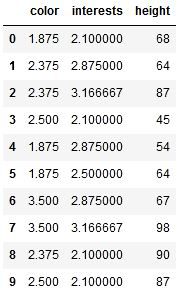GeeksforGeeks App
Open AppBrowser
Continue

# Categorical Encoding with CatBoost Encoder

Many machine learning algorithms require data to be numeric. So, before training a model, we need to convert categorical data into numeric form. There are various categorical encoding methods available. Catboost is one of them. Catboost is a target-based categorical encoder. It is a supervised encoder that encodes categorical columns according to the target value. It supports binomial and continuous targets.

Target encoding is a popular technique used for categorical encoding. It replaces a categorical feature with average value of target corresponding to that category in training dataset combined with the target probability over the entire dataset. But this introduces a target leakage since the target is used to predict the target. Such models tend to be overfitted and don’t generalize well in unseen circumstances.

A CatBoost encoder is similar to target encoding, but also involves an ordering principle in order to overcome this problem of target leakage. It uses the principle similar to the time series data validation. The values of target statistic rely on the observed history, i.e, target probability for the current feature is calculated only from the rows (observations) before it.

Categorical feature values are encoded using the following formula:TargetCount:  Sum of the target value for that particular categorical feature (upto the current one).

Prior: It is a constant value determined by (sum of target values in the whole dataset)/(total number of observations (i.e. rows) in the dataset)

FeatureCount: Total number of categorical features observed upto the current one with the same value as the current one.

With this approach, the first few observations in the dataset always have target statistics with much higher variance than the successive ones. To reduce this effect, many random permutations of the same data are used to calculate target statistics and the final encoding is calculated by averaging across these permutations. So, if the number of permutations is large enough, the final encoded values obey the following equation:TargetCount: Sum of the target value for that particular categorical feature in the whole dataset.

Prior: It is a constant value determined by (sum of target values in the whole dataset)/(total number of observations (i.e. rows) in the dataset)

FeatureCount: Total number of categorical features observed in the whole dataset with the same value as the current one.

For Example, if we have categorical feature column with values

color=[“red”, “blue”, “blue”, “green”, “red”, “red”, “black”, “black”, “blue”, “green”] and target column with values, target=[1, 2, 3, 2, 3, 1, 4, 4, 2, 3]

Then, prior will be 25/10 = 2.5

For the “red” category, TargetCount will be 1+3+1 = 5 and FeatureCount = 3

And hence, encoded value for “red” will be (5+2.5) /(3+1)=1.875

Syntax:

category_encoders.cat_boost.CatBoostEncoder(verbose=0,
cols=None, drop_invariant=False, return_df=True,
handle_unknown='value', handle_missing='value',
random_state=None, sigma=None, a=1)

Parameters:

• verbose:  Verbosity of the output, i.e., whether to print the processing output on the screen or not. 0 for no printing, positive value for printing the intermediate processing outputs.
• cols:  A list of features (columns) to be encoded. By default, it is None indicating that all columns with an object data type are to be encoded.
• drop_invariant: True means drop columns with zero variance (same value for each row). False, by default.
• return_df: True to return a pandas dataframe from transform, False will return numpy array instead. True, by default.
• handle_missing: Way of handling missing (not filled) values. error generates missing value error, return_nan returns NaN and value returns the target mean. Default is value.
• handle_unknown: Way of handling unknown (undefined) values. Options are the same as handle_missing parameter.
• sigma: It is used to decrease overfitting. Training data is added with normal distribution noise whereas testing data are left untouched. sigma is the standard deviation of that normal distribution.
• a: Float value for additive smoothing. It is needed for scenarios when attributes or data points weren’t present in the training data set but may be present in the testing data set. By default, it is set to 1. If it is not 1, the encoding equation after including this smoothing parameter takes the following form:The encoder is available as CatBoostEncoder in categorical-encodings library. This encoder works similar to scikit-learn transformers with .fit_transform(), .fit() and .transform() methods.

Example:

## Python3

 # import librariesimport category_encoders as ceimport pandas as pd  # Make datasettrain = pd.DataFrame({    'color': ["red", "blue", "blue", "green", "red",              "red", "black", "black", "blue", "green"],        'interests': ["sketching", "painting", "instruments",                  "sketching", "painting", "video games",                  "painting", "instruments", "sketching",                  "sketching"],        'height': [68, 64, 87, 45, 54, 64, 67, 98, 90, 87],        'grade': [1, 2, 3, 2, 3, 1, 4, 4, 2, 3], })  # Define train and targettarget = train[['grade']]train = train.drop('grade', axis = 1)  # Define catboost encodercbe_encoder = ce.cat_boost.CatBoostEncoder()  # Fit encoder and transform the featurescbe_encoder.fit(train, target)train_cbe = cbe_encoder.transform(train)  # We can use fit_transform() instead of fit()# and transform() separately as follows:# train_cbe = cbe_encoder.fit_transform(train,target)

Output:Dataset after Catboost encoding (train_cbe)

My Personal Notes arrow_drop_up